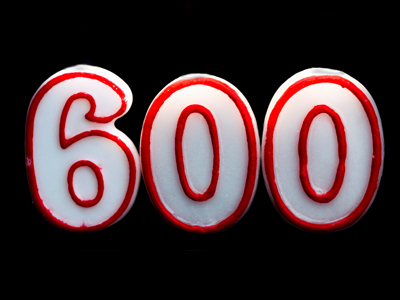The nearest 100 to 641 is 600.

# Rounding Numbers 1

This Math quiz is called 'Rounding Numbers 1' and it has been written by teachers to help you if you are studying the subject at middle school. Playing educational quizzes is a fabulous way to learn if you are in the 6th, 7th or 8th grade - aged 11 to 14.

It costs only \$12.50 per month to play this quiz and over 3,500 others that help you with your school work. You can subscribe on the page at Join Us

Rounding numbers down or up involves reducing or increasing them to the nearest ten or hundred. When we round a number we say which is the nearest ten or hundred to that number. That's easy to do with most numbers, but what about ones that end in a 5 or a 50? Well, these are always rounded up rather than down.

1.
Which of these numbers would be rounded down to the nearest 100?
134
250
879
191
Just as 5 is rounded up to 10, 50 is rounded up to 100 so 250 would not be rounded down
2.
Which is the nearest 10 to 87?
80
90
100
200
87 is only 3 less than 90
3.
Which is the nearest 10 to 33?
10
20
30
40
33 is closer to 30 than 40
4.
In which of these questions would you need to round the answer up?
A box holds 6 cakes. How many boxes will I need to hold 34 cakes?
Tickets cost £5. I have £22 how many tickets can I buy?
Candies cost 20p. I have £1. How many candies can I buy?
A pencil is 10cm. How long will 3 pencils be?
34 ÷ 6 = 5 with 4 left over. As the remaining 4 need to go in a box 6 boxes are needed
5.
Which of these numbers would be rounded down to the nearest 10?
36
25
19
91
25 is rounded up as it ends in a 5
6.
Which is the nearest 100 to 379?
100
200
300
400
379 is closer to 400 than to 300
7.
What is the nearest 100 to 641?
600
700
800
1,000
As 41 is less than 50, 641 is rounded down to 600
8.
What is the nearest 100 to 850?
700
800
900
1,000
As the number ends in 50 we always round up to the nearest 100
9.
Which is the nearest 10 to 75?
70
80
90
100
Remember that 5s are rounded up not down
10.
Why is rounding 45 more difficult?
It is half way between 40 and 50
It is a multiple of 9
It is an odd number
It is half way between 0 and 50
As 45 is half way between 40 and 50 the rule is to always round 5 up
Author:  Amanda Swift